# 17.1 Sound

 Page 1 / 3
• Define sound and hearing.
• Describe sound as a longitudinal wave.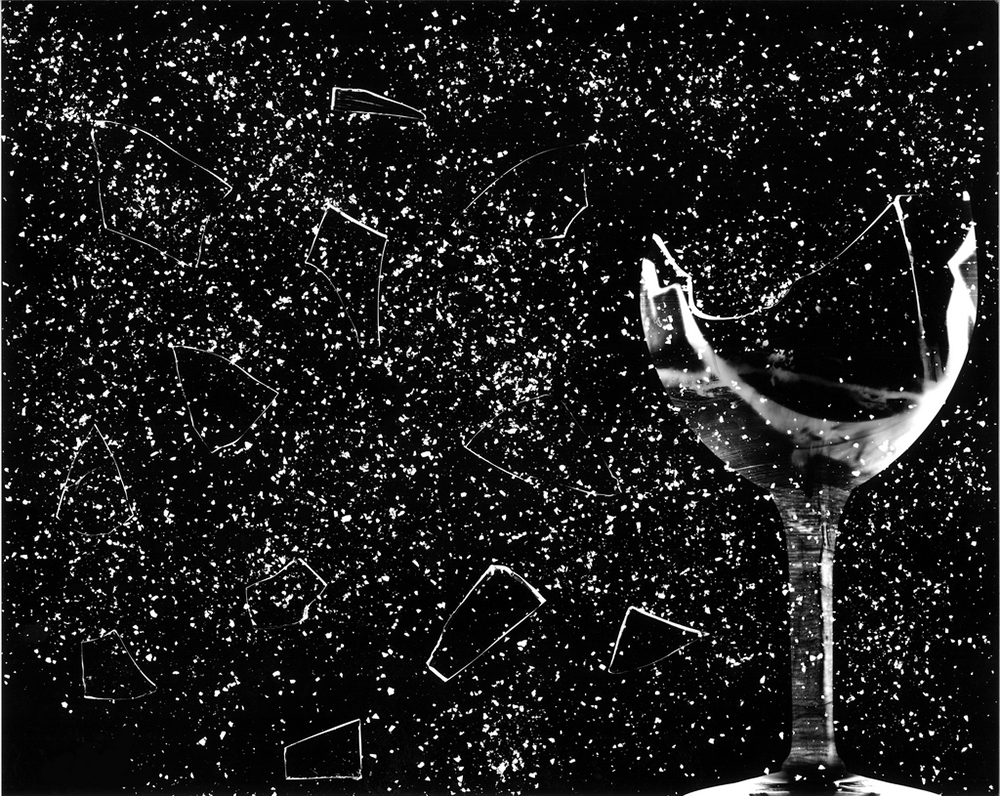This glass has been shattered by a high-intensity sound wave of the same frequency as the resonant frequency of the glass. While the sound is not visible, the effects of the sound prove its existence. (credit: ||read||, Flickr)

Sound can be used as a familiar illustration of waves. Because hearing is one of our most important senses, it is interesting to see how the physical properties of sound correspond to our perceptions of it. Hearing is the perception of sound, just as vision is the perception of visible light. But sound has important applications beyond hearing. Ultrasound, for example, is not heard but can be employed to form medical images and is also used in treatment.

The physical phenomenon of sound    is defined to be a disturbance of matter that is transmitted from its source outward. Sound is a wave. On the atomic scale, it is a disturbance of atoms that is far more ordered than their thermal motions. In many instances, sound is a periodic wave, and the atoms undergo simple harmonic motion. In this text, we shall explore such periodic sound waves.

A vibrating string produces a sound wave as illustrated in [link] , [link] , and [link] . As the string oscillates back and forth, it transfers energy to the air, mostly as thermal energy created by turbulence. But a small part of the string’s energy goes into compressing and expanding the surrounding air, creating slightly higher and lower local pressures. These compressions (high pressure regions) and rarefactions (low pressure regions) move out as longitudinal pressure waves having the same frequency as the string—they are the disturbance that is a sound wave. (Sound waves in air and most fluids are longitudinal, because fluids have almost no shear strength. In solids, sound waves can be both transverse and longitudinal.) [link] shows a graph of gauge pressure versus distance from the vibrating string.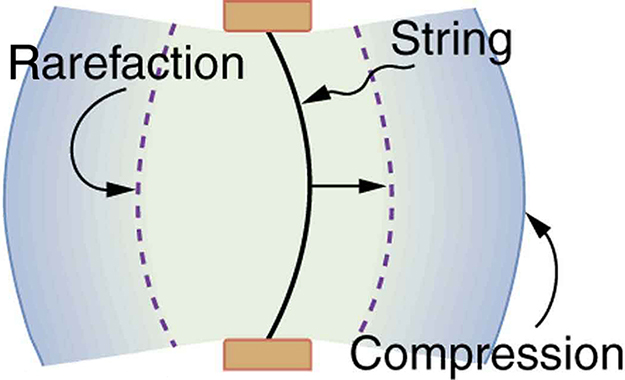A vibrating string moving to the right compresses the air in front of it and expands the air behind it.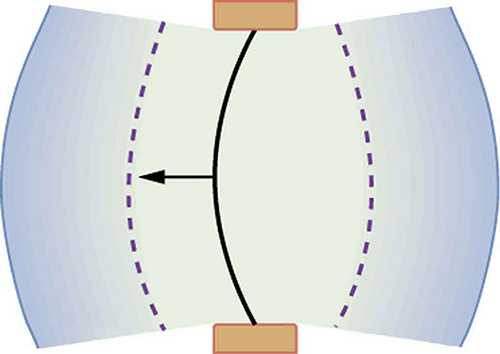As the string moves to the left, it creates another compression and rarefaction as the ones on the right move away from the string.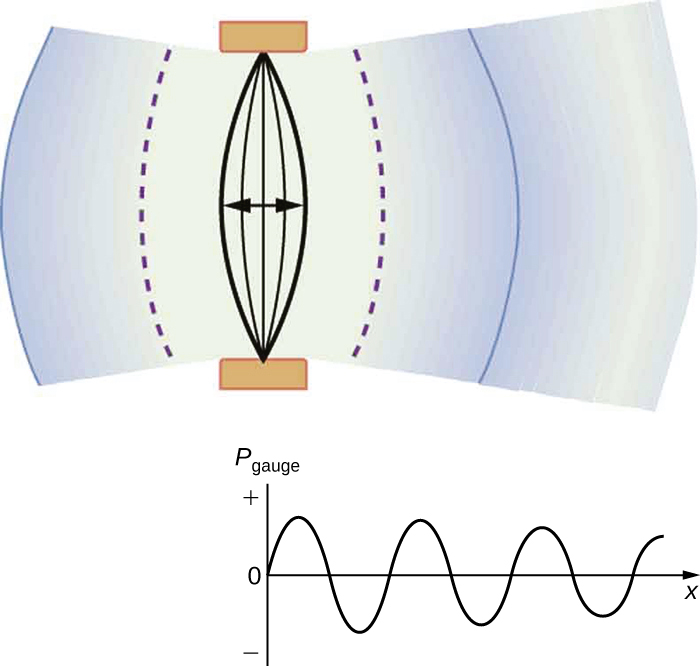After many vibrations, there are a series of compressions and rarefactions moving out from the string as a sound wave. The graph shows gauge pressure versus distance from the source. Pressures vary only slightly from atmospheric for ordinary sounds.

The amplitude of a sound wave decreases with distance from its source, because the energy of the wave is spread over a larger and larger area. But it is also absorbed by objects, such as the eardrum in [link] , and converted to thermal energy by the viscosity of air. In addition, during each compression a little heat transfers to the air and during each rarefaction even less heat transfers from the air, so that the heat transfer reduces the organized disturbance into random thermal motions. (These processes can be viewed as a manifestation of the second law of thermodynamics presented in Introduction to the Second Law of Thermodynamics: Heat Engines and Their Efficiency .) Whether the heat transfer from compression to rarefaction is significant depends on how far apart they are—that is, it depends on wavelength. Wavelength, frequency, amplitude, and speed of propagation are important for sound, as they are for all waves.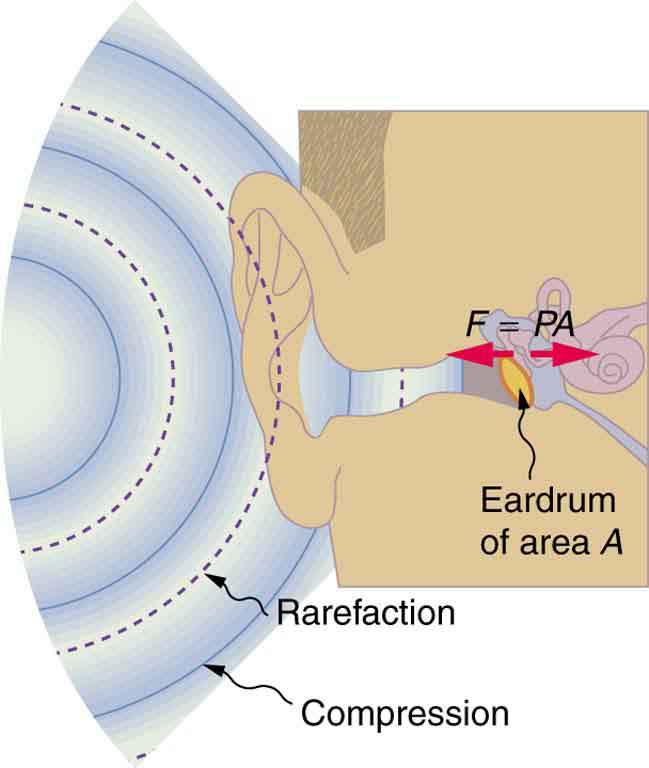Sound wave compressions and rarefactions travel up the ear canal and force the eardrum to vibrate. There is a net force on the eardrum, since the sound wave pressures differ from the atmospheric pressure found behind the eardrum. A complicated mechanism converts the vibrations to nerve impulses, which are perceived by the person.

## Phet explorations: wave interference

Make waves with a dripping faucet, audio speaker, or laser! Add a second source or a pair of slits to create an interference pattern.

## Section summary

• Sound is a disturbance of matter that is transmitted from its source outward.
• Sound is one type of wave.
• Hearing is the perception of sound.

#### Questions & Answers

What is the frictional forc e between two bodies
it is the force which always opposes the motion of the body
ZAMAN
what is a wave
wave means. A field of study
aondohemba
what are Atoms
aondohemba
is the movement back and front or up and down
sani
how ?
aondohemba
wave is a disturbance that transfers energy through matter or space with little or no associated mass.
lots
A wave is a motion of particles in disturbed medium that carry energy from one midium to another
conist
an atom is the smallest unit( particle) of an element that bares it's chemical properties
conist
what is electromagnetic induction?
conist
what's boy's law
mahmud
How is the de Broglie wavelength of electrons related to the quantization of their orbits in atoms and molecules?
How do you convert 0.0045kgcmÂ³ to the si unit?
how many state of matter do we really have like I mean... is there any newly discovered state of matter?
I only know 5: •Solids •Liquids •Gases •Plasma •Bose-Einstein condensate
Thapelo
Alright Thank you
Falana
Which one is the Bose-Einstein
James
can you explain what plasma and the I her one you mentioned
Olatunde
u can say sun or stars are just the state of plasma
Mohit
but the are more than seven
Issa
list it out I wanna know
Cristal
what the meaning of continuum
What state of matter is fire
fire is not in any state of matter...fire is rather a form of energy produced from an oxidising reaction.
Xenda
Isn`t fire the plasma state of matter?
Walter
all this while I taught it was plasma
Victor
How can you define time?
Time can be defined as a continuous , dynamic , irreversible , unpredictable quantity .
Tanaya
unpredictable? but I can say after one o'clock its going to be two o'clock predictably!
Victor
how can we define vector
mahmud
I would define it as having a magnitude (size)with a direction. An example I can think of is a car traveling at 50m/s (magnitude) going North (direction)
Hanzo
as for me guys u would say time is quantity that measures how long it takes for a specific condition to happen e.g how long it takes for the day to end or how it takes for the travelling car to cover a km.
conist
what is the relativity of physics
How do you convert 0.0045kgcm³ to the si unit?
flint
What is the formula for motion
V=u+at V²=u²-2as
flint
S=ut+½at
flint
they are eqns of linear motion
King
S=Vt
Thapelo
v=u+at s=ut+at^\2 v^=u^+2as where ^=2
King
hi
hello
King
Explain dopplers effect
Not yet learnt
Bob
Explain motion with types
Bob
Acceleration is the change in velocity over time. Given this information, is acceleration a vector or a scalar quantity? Explain.
Scalar quantity Because acceleration has only magnitude
Bob
acleration is vectr quatity it is found in a spefied direction and it is product of displcemnt
bhat
its a scalar quantity
Paul
velocity is speed and direction. since velocity is a part of acceleration that makes acceleration a vector quantity. an example of this is centripetal acceleration. when you're moving in a circular patter at a constant speed, you are still accelerating because your direction is constantly changing.
Josh
acceleration is a vector quantity. As explained by Josh Thompson, even in circular motion, bodies undergoing circular motion only accelerate because on the constantly changing direction of their constant speed. also retardation and acceleration are differentiated by virtue of their direction in
fitzgerald
respect to prevailing force
fitzgerald
What is the difference between impulse and momentum?
Manyo
Momentum is the product of the mass of a body and the change in velocity of its motion. ie P=m(v-u)/t (SI unit is kgm/s). it is literally the impact of collision from a moving body. While Impulse is the product of momentum and time. I = Pt (SI unit is kgm) or it is literally the change in momentum
fitzgerald
Or I = m(v-u)
fitzgerald
the tendency of a body to maintain it's inertia motion is called momentum( I believe you know what inertia means) so for a body to be in momentum it will be really hard to stop such body or object..... this is where impulse comes in.. the force applied to stop the momentum of such body is impulse..
Pelumi
Calculation of kinetic and potential energy
K.e=mv² P.e=mgh
Malia
K is actually 1/2 mv^2
Josh
what impulse is given to an a-particle of mass 6.7*10^-27 kg if it is ejected from a stationary nucleus at a speed of 3.2*10^-6ms²? what average force is needed if it is ejected in approximately 10^-8 s?
John
speed=velocity÷time velocity=speed×time=3.2×10^-6×10^-8=32×10^-14m/s impulse [I]=∆momentum[P]=mass×velocity=6.7×10^-27×32×10^-14=214.4×10^-41kg/ms force=impulse÷time=214.4×10^-41÷10^-8=214.4×10^-33N. dats how I solved it.if wrong pls correct me.
Melody
what is sound wave
sound wave is a mechanical longitudinal wave that transfers energy from one point to another
Ogor
its a longitudnal wave which is associted wth compresion nad rearfractions
bhat
what is power
it's also a capability to do something or act in a particular way.
Kayode
Newton laws of motion
Mike
power also known as the rate of ability to do work
Slim
power means capabilty to do work p=w/t its unit is watt or j/s it also represents how much work is done fr evry second
bhatByBy Eric CrawfordByBy Subramanian Divya